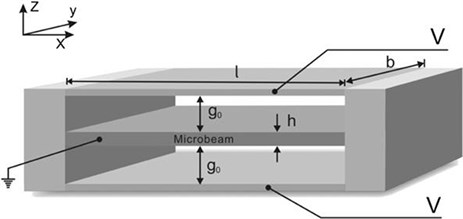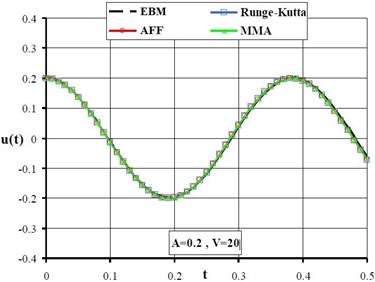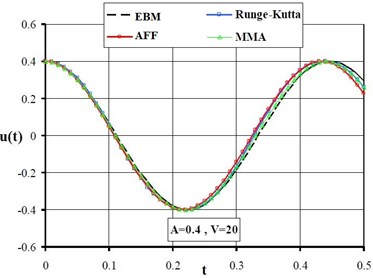Published: 15 August 2015

# Periodic solution to a nonlinear oscillator arising in micro electro mechanical system

A. Nikkar1
H. Jodeiri2
H. Tariverdian4
1Young Researchers and Elite Club, Ahar Branch, Islamic Azad University, Ahar, Iran
2Young Researchers and Elite Club, Tabriz Branch, Islamic Azad University, Tabriz, Iran
3Faculty of Civil Engineering, University of Tabriz, Tabriz, Iran
4Department of Civil Engineering, Sardroud Branch, Islamic Azad University, Sardroud, Iran
Corresponding Author:
H. Jodeiri
Views 33

#### Abstract

In this paper, a periodic solution of nonlinear oscillator arising in the micro-beam based microelectromechanical system (MEMS) has been analytically achieved. The Amplitude Frequency Formulation (AFF) and Max Min Approach (MMA) have been used. In the second method (which is called MMA), an approximate solution of the nonlinear equation can be easily deduced by finding Maximal and minimal solution thresholds of this nonlinear problem. What we understood is that both methods, works properly and scales down the deal of the work. Compare conclusions with the results from fourth order Runge-Kutta method and energy balance method (EBM) shows that obtained results are of high accuracy and convenient.

## 1. Introduction

Study micro-electro-mechanical systems (MEMS) are batch-fabricated devices and structures at a microscale level  which can be defined as a system of small dimensions (less than one cubic centimeter) fulfilling a smart function. Since its inception, MEMS technologies are of tremendous importance in various engineering fields. Because of its small size, low power consumption and high reliability, we have seen many potential applications of MEMS devices which require few mechanical components and small voltage levels for actuation actuators and sensors in aerospace, optical and biomedical engineering [2-4]. MEMS devices are generally classified according to their actuation mechanisms. Actuation mechanisms for MEMS vary depending on the suitability to the application at hand. The most common actuation mechanisms are electrostatic, pneumatic, thermal, and piezoelectric . With reported successes in applications ranging from signal filtering to chemical and mass sensing, electrostatically actuated resonant microbeam devices which form a broad class of MEMS devices due to their simplicity, as they require few mechanical components and small voltage levels for actuation  continue to be one of the most widely studied topics of the MEMS community.

Almost every natural event can be modeled with nonlinear equations; this is due to nature of the phenomena in the world. Although it is easy to find the solutions of some problems by using of computers, it is still difficult to solve nonlinear equations either numerically or analytically. Also it is very difficult to obtain the exact answer for these equations. Nonlinearity in MEMS which may cause some difficulties in computations, is made by Electrostatic actuation, large deflections and damping. According to these difficulties, some analytic techniques are available for nonlinear problems, such as MEMS [5-15]. Amplitude Frequency Formulation and Max Min approach (MMA), suggested by J. H. He. [16, 17]. Both methods are simple and effective methods to solve nonlinear oscillatory equations. In this paper, AFF and MMA are used to study a nonlinear oscillator arising in the micro-beam based MEMS where the mid plane stretching effect and distributed electrostatic force are both considered.

## 2. Problem description

Consider a clamped-clamped microbeam-based electromechanical resonator with uniform thickness $h$, length $l$, width $b$ ($b\ge 5h$), effective modulus $\stackrel{-}{E}=E/\left(1-\nu {\right)}^{2}$, Young’s modulus $E$, Poisson’s ratio $v$ and density $q$, as shown in Fig. 1 together with coordinate $oxyz$, where ${g}_{0}$ is the initial gap and $V$ the electrostatic load. The equation of motion that governs the transverse deflection is written as:

1
$\stackrel{-}{E}I\frac{{\partial }^{4}w}{\partial {x}^{4}}+\rho S\frac{{\partial }^{2}w}{\partial {t}^{2}}-\left[\stackrel{-}{N}+\frac{ES}{2l}{\underset{0}{\overset{l}{\int }}\left(\frac{\partial w}{\partial x}\right)}^{2}\right]\frac{{\partial }^{2}w}{\partial {x}^{2}}=q\left(x,t\right),$

where $S=bh$ and $\stackrel{-}{N}$ denotes the tensile or compressive axial load created by the mismatch of both thermal expansion coefficient and crystal lattice period between substrate and the thin film (microbeam). The second term on the right-hand side, $q\left(x,t\right)$, denotes the driving force per unit length, resulting from electrostatic excitation . Using the classical beam theory and taking into account of the mid-plane stretching effect as well as the distributed electrostatic force, the following dimensionless equation of motion for the microbeam can be formulated via the Galerkin method :

2
$\stackrel{¨}{u}\left({a}_{1}{u}^{4}+{a}_{2}{u}^{2}+{a}_{3}\right)+{a}_{4}u+{a}_{5}{u}^{3}+{a}_{6}{u}^{5}+{a}_{7}{u}^{7}=0,$

where:

3
${a}_{1}={\int }_{0}^{1}{\varphi }^{6}d\zeta ,$
4
${a}_{2}=-2{\int }_{0}^{1}{\varphi }^{4}d\zeta ,$
5
${a}_{3}={\int }_{0}^{1}{\varphi }^{2}d\zeta ,$
6
${a}_{4}={\int }_{0}^{1}\left(\varphi \text{'}\text{'}\text{'}\text{'}\varphi -N\varphi \text{'}\text{'}\varphi -{V}^{2}\varphi \right)d\zeta ,$
7
${a}_{5}=-{\int }_{0}^{1}\left(2\varphi \text{'}\text{'}\text{'}\text{'}{\varphi }^{3}-2N\varphi \text{'}\text{'}{\varphi }^{3}+\alpha \varphi \text{'}\text{'}\varphi {\int }_{0}^{1}\left(\varphi \text{'}{\right)}^{2}d\zeta \right)d\zeta ,$
8
${a}_{6}={\int }_{0}^{1}\left(\varphi \text{'}\text{'}\text{'}\text{'}{\varphi }^{5}-N\varphi \text{'}\text{'}{\varphi }^{5}+2\alpha \varphi \text{'}\text{'}{\varphi }^{3}{\int }_{0}^{1}\left(\varphi \text{'}{\right)}^{2}d\zeta \right)d\zeta ,$
9
${a}_{7}=-{\int }_{0}^{1}\left(\alpha {\varphi }^{\text{'}\text{'}{\varphi }^{5}{\int }_{0}^{1}\left(\varphi \text{'}{\right)}^{2}}d\zeta \right)d\zeta ,$

and

10
$\varphi =16{\xi }^{2}\left(1-{\xi }^{2}\right).$

Here, an overdot ($\bullet$) represents differentiation with respect to the time variable, while a prime ($\text{'}$) demonstrates the partial differentiation with respect to the coordinate variable $\xi$.

## 3. Basic idea of amplitude frequency formulation (AFF)

To illustrate the basic idea of AFF method, the following nonlinear oscillator is considered:

11
$\stackrel{¨}{u}+N\left(\stackrel{¨}{u},\stackrel{˙}{u},u,t\right)=0,u\left(0\right)=A,\stackrel{˙}{u}\left(0\right)=0.$

For a generalized nonlinear oscillator in Eq. (11), we use two trial functions:

12
${u}_{1}=A\mathrm{c}\mathrm{o}\mathrm{s}{\omega }_{1}t,{\omega }_{1}=1,$
13
${u}_{2}=A\mathrm{c}\mathrm{o}\mathrm{s}{\omega }_{2}t,{\omega }_{2}=\omega ,$

where $\omega$ is assumed to be the frequency of the nonlinear oscillator, Eq. (11). The residuals are:

14
${R}_{1}\left(t\right)=-\mathrm{c}\mathrm{o}\mathrm{s}t+f\left(A\mathrm{c}\mathrm{o}\mathrm{s}t\right),$
15
${R}_{2}\left(t\right)=-{\omega }^{2}\mathrm{c}\mathrm{o}\mathrm{s}\left(\omega t\right)+f\left(A\mathrm{c}\mathrm{o}\mathrm{s}\left(\omega t\right)\right).$

Fig. 1Schematics of a fixed microbeam-based electromechanical resonatorWe introduce ${R}_{11}$ and ${R}_{22}$ defined as:

16
${R}_{11}\left(t\right)=\frac{4}{{T}_{1}}{\int }_{0}^{\frac{{T}_{1}}{4}}{R}_{1}\left(t\right)\mathrm{cos}\left(t\right)dt,{T}_{1}=2\pi ,$
17
${R}_{22}\left(t\right)=\frac{4}{{T}_{2}}{\int }_{0}^{\frac{{T}_{2}}{4}}{R}_{2}\left(t\right)\mathrm{cos}\left(\omega t\right)dt,{T}_{2}=\frac{2\pi }{\omega }.$

Applying He’s frequency-amplitude formulation, we have:

18
${\omega }^{2}=\frac{{\omega }_{1}^{2}{R}_{22}\left(t\right)-{\omega }_{2}^{2}{R}_{11}\left(t\right)}{{R}_{22}\left(t\right)-{R}_{11}\left(t\right)},$

where ${\omega }_{1}=$1 and ${\omega }_{2}=\omega$.

## 4. Basic idea of max-min approach

In this section, MMA is applied to discuss the problem. To illustrate the basic idea of MMA method, the following nonlinear oscillator is considered:

19
$\stackrel{¨}{u}+N\left(\stackrel{¨}{u},\stackrel{˙}{u},u,t\right)=0,u\left(0\right)=A,\stackrel{˙}{u}\left(0\right)=0.$

In order to the fact that small parameters or linear terms are not the requirements of MMA, Eq. (18) can be approximately solved by using the MMA. Considering $a$, $b$, $c$ and $d$ as the real numbers:

20
$\frac{a}{b}

Then:

21
$\frac{a}{b}<\frac{ma+nd}{mb+nc}<\frac{d}{c},$

where $m$ and $n$ are weighting factors and $x$ is a rough approximation of:

22
$x=\frac{ma+nd}{mb+nc}.$

Eq. (19) can be rewritten in the following form:

23
$\stackrel{¨}{u}+uf\left(\stackrel{¨}{u},\stackrel{˙}{u},u,t\right)=0.$

And the frequency can be identified as follows:

24
$\frac{a}{b}<{\varpi }^{2}=\frac{ma+nd}{mb+nc}<\frac{d}{c}.$

Then:

25
$\stackrel{¨}{u}+{\varpi }^{2}u=\stackrel{¨}{u}+N\left(\stackrel{¨}{u},\stackrel{˙}{u},u,t\right)+\rho \left(\stackrel{¨}{u},\stackrel{˙}{u},u,t\right),$
26
$\rho \left(\stackrel{¨}{u},\stackrel{˙}{u},u,t\right)=0,$

here $\varpi$ can be obtained by substitution of $A\mathrm{c}\mathrm{o}\mathrm{s}\left(\varpi t\right)$ as initial assumption into Eq. (25).

## 5. Implementation of AFF

In this section, in order to assess the accuracy of He’s amplitude-frequency formulation for solving nonlinear governing equation of motion and to compare it with the other solutions, in this section we consider this method. According to He’s amplitude-frequency formulation, we choose two trial functions ${u}_{1}\left(t\right)=A\mathrm{c}\mathrm{o}\mathrm{s}t$ and ${u}_{2}\left(t\right)=A\mathrm{c}\mathrm{o}\mathrm{s}\omega t$, where $\omega$ is assumed to be the frequency of the nonlinear oscillator Eq. (2). Substituting the trial functions into Eq. (10) results in, respectively, the following residuals:

27
${R}_{1}\left(t\right)=-\left({a}_{1}{A}^{4}\mathrm{c}\mathrm{o}\mathrm{s}\left(t{\right)}^{4}+{a}_{2}{A}^{2}\mathrm{c}\mathrm{o}\mathrm{s}\left(t{\right)}^{2}+{a}_{3}\right)×A\mathrm{c}\mathrm{o}\mathrm{s}\left(t\right)+{a}_{4}A\mathrm{c}\mathrm{o}\mathrm{s}\left(t\right)+{a}_{5}{A}^{3}\mathrm{c}\mathrm{o}\mathrm{s}\left(t{\right)}^{3}$
$+{a}_{6}{A}^{5}\mathrm{c}\mathrm{o}\mathrm{s}\left(t{\right)}^{5}+{a}_{7}{A}^{7}\mathrm{c}\mathrm{o}\mathrm{s}\left(t{\right)}^{7},$
28
${R}_{2}\left(t\right)=-\left({a}_{1}{A}^{4}\mathrm{c}\mathrm{o}\mathrm{s}\left(\omega t{\right)}^{4}+{a}_{2}{A}^{2}\mathrm{c}\mathrm{o}\mathrm{s}\left(\omega t{\right)}^{2}+{a}_{3}\right)×A\mathrm{c}\mathrm{o}\mathrm{s}\left(\omega t{\right)}^{2}{\omega }^{2}+{a}_{4}A\mathrm{c}\mathrm{o}\mathrm{s}\left(\omega t\right)$
$+{a}_{5}{A}^{3}\mathrm{c}\mathrm{o}\mathrm{s}\left(\omega t{\right)}^{3}+{a}_{6}{A}^{5}\mathrm{c}\mathrm{o}\mathrm{s}\left(\omega t{\right)}^{5}+{a}_{7}{A}^{7}\mathrm{c}\mathrm{o}\mathrm{s}\left(\omega t{\right)}^{7}.$

We introduce ${R}_{11}$ and ${R}_{22}$ defined as:

29
${R}_{11}\left(t\right)=\frac{4}{{T}_{1}}{\int }_{0}^{\frac{{T}_{1}}{4}}{R}_{1}\left(t\right)\mathrm{c}\mathrm{o}\mathrm{s}\left(t\right)dt=\frac{1}{128}A×\left(40{a}_{6}{A}^{4}-40{a}_{1}{A}^{4}-48{a}_{2}{A}^{2}+48{a}_{5}{A}^{4}$
$\mathrm{}\mathrm{}\mathrm{}\mathrm{}+35{a}_{7}{A}^{6}+64{a}_{4}-64{a}_{3}\right),\mathrm{}\mathrm{}\mathrm{}{T}_{1}=2\pi ,$
30
${R}_{22}\left(t\right)=\frac{4}{{T}_{2}}{\int }_{0}^{\frac{{T}_{2}}{4}}{R}_{2}\left(t\right)\mathrm{cos}\left(\omega t\right)dt=\frac{1}{128}A×\left(48{a}_{5}{A}^{2}+40{a}_{6}{A}^{4}+35{a}_{7}{A}^{6}+64{a}_{4}$
$-64{\omega }^{2}{a}_{3}-40{A}^{4}{\omega }^{2}{a}_{1}-48{A}^{2}{\omega }^{2}{a}_{2}\right),{T}_{2}=\frac{2\pi }{\omega }.$

Applying He’s frequency-amplitude formulation, we have:

31
${\omega }^{2}=\frac{{\omega }_{1}^{2}{R}_{22}\left(t\right)-{\omega }_{2}^{2}{R}_{11}\left(t\right)}{{R}_{22}\left(t\right)-{R}_{11}\left(t\right)},$

where ${\omega }_{1}=$1 and ${\omega }_{2}=\omega$. Finally, the amplitude-depended frequency can be approximated as:

32
${\omega }_{AFF}=\frac{\sqrt{2}}{4}\frac{\sqrt{64{a}_{4}+48{a}_{5}{A}^{2}+40{a}_{6}{A}^{4}+35{a}_{7}{A}^{6}}}{\sqrt{5{a}_{1}{A}^{4}+6{a}_{2}{A}^{2}+8{a}_{3}}}.$

33
$u\left(t\right)=A\mathrm{c}\mathrm{o}\mathrm{s}\left(\frac{\sqrt{2}}{4}\frac{\sqrt{64{a}_{4}+48{a}_{5}{A}^{2}+40{a}_{6}{A}^{4}+35{a}_{7}{A}^{6}}}{\sqrt{5{a}_{1}{A}^{4}+6{a}_{2}{A}^{2}+8{a}_{3}}}t\right).$

## 6. Application of MMA

In this section, the MMA is applied to solve nonlinear sector. Eq. (2) can be rewritten in the following form:

34
$\stackrel{¨}{u}+\left(\frac{{a}_{1}}{{a}_{3}}{u}^{3}\stackrel{¨}{u}+\frac{{a}_{2}}{{a}_{3}}u\stackrel{¨}{u}+\frac{{a}_{4}}{{a}_{3}}+\frac{{a}_{5}}{{a}_{3}}{u}^{2}+\frac{{a}_{6}}{{a}_{3}}{u}^{4}+\frac{{a}_{7}}{{a}_{3}}{u}^{6}\right)u=0.$

In addition, boundary conditions are considered. Here, if $u\left(t\right)=A\mathrm{c}\mathrm{o}\mathrm{s}\left(\varpi t\right)$ be chosen as a trial function, then:

35
$\frac{{a}_{4}}{{a}_{3}}<\frac{{a}_{1}}{{a}_{3}}{u}^{3}\stackrel{¨}{u}+\frac{{a}_{2}}{{a}_{3}}u\stackrel{¨}{u}+\frac{{a}_{4}}{{a}_{3}}+\frac{{a}_{5}}{{a}_{3}}{u}^{2}+\frac{{a}_{6}}{{a}_{3}}{u}^{4}+\frac{{a}_{7}}{{a}_{3}}{u}^{6}$
$<\frac{-{a}_{1}{A}^{4}{\varpi }^{2}-{a}_{2}{A}^{2}{\varpi }^{2}+{a}_{4}+{a}_{5}{A}^{2}+{a}_{6}{A}^{4}+{a}_{7}{A}^{6}}{{a}_{3}},$

where ${a}_{4}$ is the minimum and $-{\mathrm{a}}_{1}{A}^{4}{\varpi }^{2}-{a}_{2}{A}^{2}{\varpi }^{2}+{a}_{4}+{a}_{5}{A}^{2}+{a}_{6}{A}^{4}+{a}_{7}{A}^{6}$ is the maximum value of $\frac{{a}_{1}}{{a}_{3}}{u}^{3}\stackrel{¨}{u}+\frac{{a}_{2}}{{a}_{3}}u\stackrel{¨}{u}+\frac{{a}_{4}}{{a}_{3}}+\frac{{a}_{5}}{{a}_{3}}{u}^{2}+\frac{{a}_{6}}{{a}_{3}}{u}^{4}+\frac{{a}_{7}}{{a}_{3}}{u}^{6}$.

Here the frequency value can be identified as follows:

36
${\varpi }^{2}=\frac{n}{\left(m+n\right)}\cdot \frac{-{a}_{1}{A}^{4}{\varpi }^{2}-{a}_{2}{A}^{2}{\varpi }^{2}+{a}_{4}+{a}_{5}{A}^{2}+{a}_{6}{A}^{4}+{a}_{7}{A}^{6}}{{a}_{3}}+\frac{m}{\left(m+n\right)}\cdot \frac{{a}_{4}}{{a}_{3}}$
$=\frac{k\left(-{a}_{1}{A}^{4}{\varpi }^{2}-{a}_{2}{A}^{2}{\varpi }^{2}+{a}_{5}{A}^{2}+{a}_{6}{A}^{4}+{a}_{7}{A}^{6}\right)}{{a}_{3}}+\frac{{a}_{4}}{{a}_{3}},$

where $m$ and $n$ are weighting factors, and $k=n/\left(m+n\right)$. So the frequency can be approximated as follows:

37
$\varpi =\sqrt{\frac{k\left(-{a}_{1}{A}^{4}{\varpi }^{2}-{a}_{2}{A}^{2}{\varpi }^{2}+{a}_{5}{A}^{2}+{a}_{6}{A}^{4}+{a}_{7}{A}^{6}\right)}{{a}_{3}}+\frac{{a}_{4}}{{a}_{3}}}.$

Then Eq. (24) can be rewritten as follows:

38
$\stackrel{¨}{u}+{\varpi }^{2}u=\stackrel{¨}{u}+\left(\frac{{a}_{1}}{{a}_{3}}{u}^{3}\stackrel{¨}{u}+\frac{{a}_{2}}{{a}_{3}}u\stackrel{¨}{u}+\frac{{a}_{4}}{{a}_{3}}+\frac{{a}_{5}}{{a}_{3}}{u}^{2}+\frac{{a}_{6}}{{a}_{3}}{u}^{4}+\frac{{a}_{7}}{{a}_{3}}{u}^{6}\right)u+\rho ,$

where:

39
$\rho ={a}_{3}\left(\frac{{a}_{1}}{{a}_{3}}{u}^{3}\stackrel{¨}{u}+\frac{{a}_{2}}{{a}_{3}}u\stackrel{¨}{u}+\frac{{a}_{4}}{{a}_{3}}+\frac{{a}_{5}}{{a}_{3}}{u}^{2}+\frac{{a}_{6}}{{a}_{3}}{u}^{4}+\frac{{a}_{7}}{{a}_{3}}{u}^{6}\right)u$
$+\left(-k{a}_{1}{A}^{4}{\varpi }^{2}-k{a}_{2}{A}^{2}{\varpi }^{2}+{a}_{4}+k{a}_{5}{A}^{2}+k{a}_{7}{A}^{6}\right)u.$

Combining $u\left(t\right)=A\mathrm{c}\mathrm{o}\mathrm{s}\left(\varpi t\right)$ with $\rho$, and using Fourier expansion series gives:

40
$\rho \left(\varpi t\right)=\sum _{n=0}^{\infty }{\delta }_{2n+1}\mathrm{c}\mathrm{o}\mathrm{s}\left[\left(2n+1\right)\varpi t\right]={\delta }_{1}\mathrm{c}\mathrm{o}\mathrm{s}\varpi t+{\delta }_{3}\mathrm{c}\mathrm{o}\mathrm{s}3\varpi t+...\cong {\delta }_{1}\mathrm{c}\mathrm{o}\mathrm{s}\left(\varpi t\right),$
41
${\delta }_{1}=\left(\frac{4}{\pi }{\int }_{0}^{\frac{\pi }{2}}\rho \left(\varphi \right)\mathrm{c}\mathrm{o}\mathrm{s}\left(\varphi \right)d\varphi \right)=\frac{8}{315\pi }\left(-105k{a}_{1}{A}^{4}{\varpi }^{2}-105k{a}_{2}{A}^{2}{\varpi }^{2}+210{a}_{4}$
$+105k{a}_{5}{A}^{2}+105k{a}_{6}{A}^{4}+105k{a}_{7}{A}^{6}-72{a}_{1}{A}^{4}{\varpi }^{2}-84{a}_{2}{A}^{2}{\varpi }^{2}+84{a}_{5}{A}^{2}$
$+72{a}_{6}{A}^{4}+64{a}_{7}{A}^{6}\right).$

In order to avoid secular term, the following equation can be set:

42
${\delta }_{1}=0.$

Then the following equation can be obtained:

43
$k=\frac{2}{105}\frac{-36{a}_{1}{A}^{4}{\varpi }^{2}-42{a}_{2}{A}^{2}\varpi +105{a}_{4}+42{a}_{5}{A}^{2}+36{a}_{6}{A}^{4}+32{a}_{7}{A}^{6}}{{A}^{2}\left(-{A}^{2}{a}_{1}{\varpi }^{2}-{a}_{2}{\varpi }^{2}+{a}_{5}+{A}^{2}{a}_{6}+{A}^{4}{a}_{7}\right)}.$

Substituting Eq. (42) into Eq. (36), yields:

44
${\varpi }_{MMA}=\frac{\sqrt{3}}{3}\sqrt{\frac{84{a}_{5}{A}^{2}+64{a}_{7}{A}^{6}+72{a}_{6}{A}^{4}+105{a}_{4}}{24{a}_{1}{A}^{4}+35{a}_{3}+28{a}_{2}{A}^{2}}}.$

The answers of AFF method and max-min approach are plotted in Fig. 2. For comparison, results of energy balance  and numerical fourth-order Runge-Kutta method are plotted in these figures too. Herein the values of parameters are taken as $N=$ 15, $\alpha =\mathrm{}$10.

Fig. 2The results of MMA, AFF, EBM and Runge-Kutta method for A= 0.2 and 0.4, N= 15, α= 10## 7. Conclusion

In this paper, the main purpose was to illustrate the application of amplitude frequency formulation (AFF) and max-min approach (MMA) in solving nonlinear oscillator arising in the microbeam based MEMS. Also, the capabilities and facile applications of these methods have been demonstrated in comparison with the numerical solution and energy balance method. By intensifying the value of voltage and initial condition A, it has been considered that the disagreement between the answers obtained by AFF, MMA and Runge-Kutta is very insignificant which indicates that these methods provide highly precise answers for nonlinear equations.